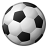cancel
Showing results for
Did you mean:Creator III

## Pivot tables

Hi folks,

This question is with respect to Qlikview. Please consider the below table.

 Date Open Closed 17-10-2018 30 15 18-10-2018 22 54 19-10-2018 32 14 20-10-2018 11 15 Variance -21 1

I have two dimensions DATE and Indicator (Open and Closed ) and one expression count. I need a variance row in the last row that is the difference between two recent dates, in this case 20th oct and 19th oct. How can i get that variance row with respect two the most recent dates and that too in the pivot table itself.

Thanks for the help!

1 Solution

Accepted SolutionsMVP

My bad with the syntax (fixed now)... try this for your sample

=If(Dimensionality() = 0,

Count({<Date = {"\$(=Date(Max(Date)))"}>} Indicator)-Count({<Date = {"\$(=Date(Max(Date, 2)))"}>} Indicator),

Count(Indicator))

4 RepliesMVP

May be like this

If(Dimensionality() = 0, Count({<Date = {"\$(=Date(Max(Date)))"}>} Measure)-Count({<Date = {"\$(=Date(Max(Date, 2)))"}>} Measure), Count(Measure))

and then make sure to enable subtotals from the presentation tabCreator III
Author

Hey Sunny,

Thanks for the quick reply, but im not still not getting the required output. Im attaching a sample file.MVP

My bad with the syntax (fixed now)... try this for your sample

=If(Dimensionality() = 0,

Count({<Date = {"\$(=Date(Max(Date)))"}>} Indicator)-Count({<Date = {"\$(=Date(Max(Date, 2)))"}>} Indicator),

Count(Indicator))Creator III
Author

This working perfectly. I was not sure we could have user Dimensionality() in such a way.

Thanks again!Community Browser